# Congruences for $${\varvec{q}}$$-Binomial Coefficients

## Abstract

We discuss q-analogues of the classical congruence $$\left( {\begin{array}{c}ap\\ bp\end{array}}\right) \equiv \left( {\begin{array}{c}a\\ b\end{array}}\right) \pmod {p^3}$$, for primes $$p>3$$, as well as its generalisations. In particular, we prove related congruences for (q-analogues of) integral factorial ratios.

## Introduction

For a non-negative integer a, a standard q-environment includes the q-numbers $$[a]=[a]_q=(1-q^a)/(1-q)\in {\mathbb {Z}}[q]$$, the q-factorials $$[a]!=\cdots [a]\in {\mathbb {Z}}[q]$$ and the q-binomial coefficients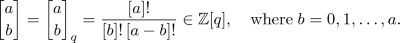One also adopts the cyclotomic polynomials

\begin{aligned} \Phi _n(q)=\prod _{\begin{array}{c} j=1\\ (j,n)=1 \end{array}}^n(q-e^{2\pi ij/n})\in {\mathbb {Z}}[q] \end{aligned}

as q-analogues of prime numbers, because these are the only factors of the q-numbers which are irreducible over $${\mathbb {Q}}$$.

Arithmetically significant relations often possess several q-analogues. While looking for q-extensions of the classical (Wolstenholme–Ljunggren) congruence

\begin{aligned} \left( {\begin{array}{c}ap\\ bp\end{array}}\right) \equiv \left( {\begin{array}{c}a\\ b\end{array}}\right) \pmod {p^3} \quad \text {for any prime}\; p>3, \end{aligned}
(1.1)

more precisely, at a ‘q-microscope setup’ (when q-congruences for truncated hypergeometric sums are read off from the asymptotics of their non-terminating versions, usually equipped with extra parameters, at roots of unity, see ) for Straub’s q-congruence [8, 9, Theorem 2.2],(1.2)

this author accidentally arrived at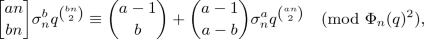(1.3)

where the notation

\begin{aligned} \sigma _n=(-1)^{n-1} \end{aligned}

is implemented. Notice that the expression on the right-hand side is a sum of two q-monomials. The q-congruence (1.3) may be compared with another q-extension of (1.1),(1.4)

for any $$n>1$$. This is given by Andrews in  for primes $$n=p>3$$ only; though proved modulo $$\Phi _p(q)^2$$, a complimentary result from  demonstrates that (1.1) in its full modulo $$p^3$$ strength can be derived from (1.4). More directly, Pan  shows that (1.4) can be generalised further to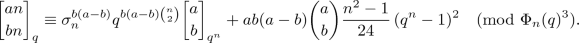(1.5)

It is worth mentioning that the transition from $$\Phi _n(q)^2$$ to $$\Phi _n(q)^3$$ (or, from $$p^2$$ to $$p^3$$) is significant because the former has a simple combinatorial proof (resulting from the q-Chu–Vandermonde identity) whereas no combinatorial proof is known for the latter.

Since $$q^{\left( {\begin{array}{c}bn\\ 2\end{array}}\right) }\sim \sigma _n^b$$ as $$q\rightarrow \zeta$$, a primitive n-th root of unity, the congruence (1.3) is seen to be an extension of the trivial (q-Lucas) congruenceThe principal goal of this note is to provide a modulo $$\Phi _n(q)^4$$ extension of (1.3) (see Lemma 2.1 below) as well as to use the result for extending the congruences (1.2) and (1.5). In this way, our theorems provide two q-extensions of the congruence

\begin{aligned} \left( {\begin{array}{c}ap\\ bp\end{array}}\right) \equiv \left( {\begin{array}{c}a\\ b\end{array}}\right) +ab(a-b)\left( {\begin{array}{c}a\\ b\end{array}}\right) p\sum _{k=1}^{p-1}\frac{1}{k}\pmod {p^4} \quad \text {for prime}~ p>3. \end{aligned}

The latter can be continued further to higher powers of primes , and our ‘mechanical’ approach here suggests that one may try—with a lot of effort!—to deduce corresponding q-analogues.

### Theorem 1.1

The congruence(1.6)

holds modulo $$\Phi _n(q)^4$$ for any $$n>1$$.

### Theorem 1.2

For any $$n>1$$, we have the congruence(1.7)

We point out that a congruence $$A_1(q)\equiv A_2(q)\pmod {P(q)}$$ for rational functions $$A_1(q),A_2(q)\in {\mathbb {Q}}(q)$$ and a polynomial $$P(q)\in {\mathbb {Q}}[q]$$ is understood as follows: the polynomial P(q) is relatively prime with the denominators of $$A_1(q)$$ and $$A_2(q)$$, and P(q) divides the numerator A(q) of the difference $$A_1(q)-A_2(q)$$. The latter is equivalent to the condition that for each zero $$\alpha \in {\mathbb {C}}$$ of P(q) of multiplicity k, the polynomial $$(q-\alpha )^k$$ divides A(q) in $${\mathbb {C}}[q]$$; in other words, $$A_1(q)-A_2(q)=O\bigl ((q-\alpha )^k\bigr )$$ as $$q\rightarrow \alpha$$. This latter interpretation underlies our argument in proving the results. For example, the congruence (1.3) can be established by verifying that(1.8)

when $$q=\zeta (1-\varepsilon )$$ and $$\zeta$$ is any primitive n-th root of unity.

Our approach goes in line with  and shares similarities with the one developed by Gorodetsky in , who reads off the asymptotic information of binomial sums at roots of unity through the q-Gauss congruences. It does not seem straightforward to us but Gorodetsky’s method may be capable of proving Theorems 1.1 and 1.2. Furthermore, the part [4, Sect. 2.3] contains a survey on q-analogues of (1.1).

After proving an asymptotical expansion for q-binomial coefficients at roots of unity in Sect. 2 [essentially, the $$O(\varepsilon ^4)$$-extension of (1.8)], we perform a similar asymptotic analysis for q-harmonic sums in Sect. 3. The information gathered is then applied in Sect. 4 to proving Theorems 1.1 and 1.2. Finally, in Sect. 5, we generalise the congruences (1.2) and (1.5) in a different direction, to integral factorial ratios.

## Expansions of q-Binomials at Roots of Unity

This section is exclusively devoted to an asymptotical result, which forms the grounds of our later arithmetic analysis. We moderate its proof by highlighting principal ingredients (and difficulties) of derivation and leaving some technical details to the reader.

### Lemma 2.1

Let $$\zeta$$ be a primitive n-th root of unity. Then, as $$q=\zeta (1-\varepsilon )\rightarrow \zeta$$ radially,(2.1)

where

\begin{aligned} \rho _0(a,n)&=\frac{3(an-1)^2-an^2-1}{24},\\ \rho _1(a,b,n)&=\frac{abn^2(an-1)(an-n-2)+(an+2)(an-1)^2(an-3)+an^2+a+2}{48} \end{aligned}

and

\begin{aligned} S_{n-1}(q)=\frac{1}{2}\sum _{k=1}^{n-1}\frac{kq^k((k+1)q^k+k-1)}{(1-q^k)^3}. \end{aligned}

### Proof

It follows from the q-binomial theorem [3, Chap. 10] that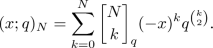(2.2)

Taking $$N=an$$, for a primitive n-th root of unity $$\zeta =\zeta _n$$, we have(2.3)

When $$q=\zeta (1-\varepsilon )$$, we get $${\mathrm d}/{\mathrm d}\varepsilon =-\zeta \,({\mathrm d}/{\mathrm d}q)$$. If

\begin{aligned} f(q)=(x;q)_{an} \quad \text {and}\quad g(q)=\frac{{\mathrm d}}{{\mathrm d}q}\log f(q)=-\sum _{\ell =1}^{an-1}\frac{\ell q^{\ell -1}x}{1-q^\ell x}, \end{aligned}

then $$f(q)|_{\varepsilon =0}=(1-x^n)^a$$ and

\begin{aligned} \frac{{\mathrm d}f}{{\mathrm d}q}=fg, \quad \frac{{\mathrm d}^2f}{{\mathrm d}q^2}=f\biggl (g^2+\frac{{\mathrm d}g}{{\mathrm d}q}\biggr ), \quad \frac{{\mathrm d}^3f}{{\mathrm d}q^3}=f\biggl (g^3+3g\frac{{\mathrm d}g}{{\mathrm d}q}+\frac{{\mathrm d}^2g}{{\mathrm d}q^2}\biggr ). \end{aligned}

In particular,

\begin{aligned} \frac{{\mathrm d}f}{{\mathrm d}\varepsilon }\bigg |_{\varepsilon =0}&=(1-x^n)^a\sum _{\ell =1}^{an-1}\frac{\ell \zeta ^\ell x}{1-\zeta ^\ell x}, \\ \frac{{\mathrm d}^2f}{{\mathrm d}\varepsilon ^2}\bigg |_{\varepsilon =0}&=(1-x^n)^a\biggl (\biggl (\sum _{\ell =1}^{an-1}\frac{\ell \zeta ^\ell x}{1-\zeta ^\ell x}\biggr )^2 -\sum _{\ell =1}^{an-1}\biggl (\frac{\ell ^2\zeta ^{2\ell }x^2}{(1-\zeta ^\ell x)^2}+\frac{\ell (\ell -1)\zeta ^\ell x}{1-\zeta ^\ell x}\biggr )\biggr ) \end{aligned}

and

\begin{aligned} \frac{{\mathrm d}^3f}{{\mathrm d}\varepsilon ^3}\bigg |_{\varepsilon =0}&=(1-x^n)^a\biggl (\biggl (\sum _{\ell =1}^{an-1}\frac{\ell \zeta ^\ell x}{1-\zeta ^\ell x}\biggr )^3 \\&\quad -3\sum _{\ell =1}^{an-1}\frac{\ell \zeta ^\ell x}{1-\zeta ^\ell x} \sum _{\ell =1}^{an-1}\biggl (\frac{\ell ^2\zeta ^{2\ell }x^2}{(1-\zeta ^\ell x)^2}+\frac{\ell (\ell -1)\zeta ^\ell x}{1-\zeta ^\ell x}\biggr ) \\&\quad +\sum _{\ell =1}^{an-1}\biggl (\frac{2\ell ^3\zeta ^{3\ell }x^3}{(1-\zeta ^\ell x)^3}+\frac{3\ell ^2(\ell -1)\zeta ^{2\ell }x^2}{(1-\zeta ^\ell x)^2}+\frac{\ell (\ell -1)(\ell -2)\zeta ^\ell x}{1-\zeta ^\ell x}\biggr )\biggr ). \end{aligned}

Now observe the following summation formulae:

\begin{aligned} \frac{1}{n}\sum _{j=1}^n\frac{x}{1-x}\bigg |_{x\mapsto \zeta ^jx}&=\frac{x^n}{1-x^n}, \\ \frac{1}{n}\sum _{j=1}^n\biggl (\frac{x}{1-x}\biggr )^2\bigg |_{x\mapsto \zeta ^jx}&=\frac{nx^n}{(1-x^n)^2}-\frac{x^n}{1-x^n}, \\ \frac{1}{n}\sum _{j=1}^n\frac{x}{1-x}\,\frac{\zeta ^kx}{1-\zeta ^kx}\bigg |_{x\mapsto \zeta ^jx}&=-\frac{x^n}{1-x^n} \quad \text {for}\; k\not \equiv 0\pmod n, \\ \frac{1}{n}\sum _{j=1}^n\biggl (\frac{x}{1-x}\biggr )^3\bigg |_{x\mapsto \zeta ^jx}&=\frac{n^2x^n(1+x^n)}{2(1-x^n)^3}-\frac{3nx^n}{2(1-x^n)^2}+\frac{x^n}{1-x^n}, \\ \frac{1}{n}\sum _{j=1}^n\frac{x}{1-x}\,\frac{\zeta ^kx}{1-\zeta ^kx}\,\frac{\zeta ^\ell x}{1-\zeta ^\ell x}\bigg |_{x\mapsto \zeta ^jx}&=\frac{x^n}{1-x^n} \\&\qquad \text {for}\; k\not \equiv 0,\; \ell \not \equiv 0,\; k\not \equiv \ell \pmod n, \end{aligned}

and

\begin{aligned} \frac{1}{n}\sum _{j=1}^n\biggl (\frac{x}{1-x}\biggr )^2\frac{\zeta ^kx}{1-\zeta ^kx}\bigg |_{x\mapsto \zeta ^jx}&=\frac{nx^n}{(1-x^n)^2}\,\frac{\zeta ^k}{1-\zeta ^k}+\frac{x^n}{1-x^n} \\&\quad \text {for}~ k\not \equiv 0\pmod n. \end{aligned}

Implementing this information in (2.3), we obtain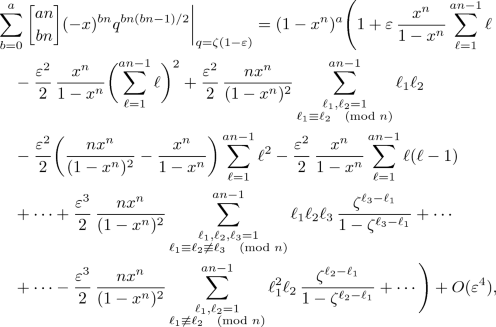where we intentionally omit all ordinary $$\varepsilon ^3$$-terms—those that sum up to polynomials in a and n multiplied by powers of $$x^n/(1-x^n)$$, like the ones appearing as $$\varepsilon$$- and $$\varepsilon ^2$$-terms. The exceptional $$\varepsilon ^3$$-summands are computed separately:

\begin{aligned} \sum _{\begin{array}{c} \ell _1,\ell _2,\ell _3=1\\ \ell _1\equiv \ell _2\not \equiv \ell _3\pmod n \end{array}}^{an-1}\ell _1\ell _2\ell _3\,\frac{\zeta ^{\ell _3-\ell _1}}{1-\zeta ^{\ell _3-\ell _1}} =-a^3\sum _{k=1}^{n-1}\frac{k\zeta ^k((k+1)\zeta ^k+k-1)}{(1-\zeta ^k)^3} \\ \quad -\frac{a^3n(n-1)(3an(an-1)(an-2)+n^2(an-2a-1)-2)}{48} \end{aligned}

and

\begin{aligned} \sum _{\begin{array}{c} \ell _1,\ell _2=1\\ \ell _1\not \equiv \ell _2\pmod n \end{array}}^{an-1} \ell _1^2\ell _2\,\frac{\zeta ^{\ell _2-\ell _1}}{1-\zeta ^{\ell _2-\ell _1}} =-a^2\sum _{k=1}^{n-1}\frac{k\zeta ^k((k+1)\zeta ^k+k-1)}{(1-\zeta ^k)^3} \\ \quad -\frac{a^2n(n-1)(an(an-1)(2an-3)-an^2-1)}{24}. \end{aligned}

The finale of our argument is comparison of the coefficients of powers of $$x^n$$ on both sides of the relation obtained; this way we arrive at the asymptotics in (2.1). $$\square$$

## A q-Harmonic Sum

Again, the notation $$\zeta$$ is reserved for a primitive n-th root of unity. For the sum

\begin{aligned} H_{n-1}(q)=\sum _{k=1}^{n-1}\frac{q^k}{1-q^k}, \end{aligned}

we have

\begin{aligned} \frac{{\mathrm d}H_{n-1}}{{\mathrm d}q}&=\sum _{k=1}^{n-1}\frac{kq^{k-1}}{(1-q^k)^2}, \\ \frac{{\mathrm d}^2H_{n-1}}{{\mathrm d}q^2}&=\sum _{k=1}^{n-1}\frac{kq^{k-2}((k+1)q^k+k-1)}{(1-q^k)^3}=2q^{-2}S_{n-1}(q), \end{aligned}

where $$S_{n-1}(q)$$ is defined in Lemma 2.1. It follows that, for $$q=\zeta (1-\varepsilon )$$,

\begin{aligned} H_{n-1}(q)&=\sum _{k=1}^{n-1}\frac{\zeta ^k}{1-\zeta ^k} -\varepsilon \sum _{k=1}^{n-1}\frac{k\zeta ^k}{(1-\zeta ^k)^2}\end{aligned}
(3.1)
\begin{aligned}&\quad +\frac{\varepsilon ^2}{2}\sum _{k=1}^{n-1}\frac{k\zeta ^k((k+1)\zeta ^k+k-1)}{(1-\zeta ^k)^3} +O(\varepsilon ^3) \nonumber \\&=-\frac{n-1}{2}+\frac{(n^2-1)n}{24}\,\varepsilon +S_{n-1}(\zeta )\varepsilon ^2 +O(\varepsilon ^3)\nonumber \\&=-\frac{n-1}{2}-\frac{n^2-1}{24}\,(q^n-1)+\frac{(n-1)(n^2-1)}{48n}(q^n-1)^2 \nonumber \\&\quad +\frac{1}{n^2}S_{n-1}(\zeta )(q^n-1)^2 +O(\varepsilon ^3) \end{aligned}
(3.2)

as $$\varepsilon \rightarrow 0$$, where we use

\begin{aligned} \varepsilon =-\frac{1}{n}\,(q^n-1)+\frac{n-1}{2n^2}\,(q^n-1)^2+O(\varepsilon ^3) \quad \text {as}~ \varepsilon \rightarrow 0. \end{aligned}

The latter asymptotics implies that

\begin{aligned} \sum _{k=1}^{n-1}\frac{q^k}{1-q^k}&\equiv -\frac{n-1}{2}-\frac{n^2-1}{24}\,(q^n-1)+\frac{(n-1)(n^2-1)}{48n}(q^n-1)^2 \\&\quad +\frac{(q^n-1)^2}{2n^2}\sum _{k=1}^{n-1}\frac{kq^k((k+1)q^k+k-1)}{(1-q^k)^3} \pmod {\Phi _n(q)^3}, \end{aligned}

which may be viewed as an extension of

\begin{aligned} \sum _{k=1}^{n-1}\frac{q^k}{1-q^k} \equiv -\frac{n-1}{2}-\frac{n^2-1}{24}\,(q^n-1) \pmod {\Phi _n(q)^2} \end{aligned}

recorded, for example, in .

A different consequence of (3.2) is the following fact.

### Lemma 3.1

The term $$\varepsilon ^2S_{n-1}(\zeta )$$ appearing in the expansion (2.1) can be replaced with

\begin{aligned} H_{n-1}(q)+\frac{n-1}{2}+\frac{n^2-1}{24}\,(q^n-1)-\frac{(n-1)(n^2-1)}{48n}(q^n-1)^2+O(\varepsilon ^3) \end{aligned}

when $$q=\zeta (1-\varepsilon )$$ and $$\varepsilon \rightarrow 0$$.

## Proof of the Theorems

To prove Theorems 1.1 and 1.2, we need to produce ‘matching’ asymptotics for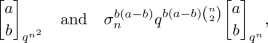respectively. These happen to be easier than that from Lemma 2.1 because $$q^{n^2}=(1-\varepsilon )^{n^2}$$ and $$q^n=(1-\varepsilon )^n$$ do not depend on the choice of primitive n-th root of unity $$\zeta$$ when $$q=\zeta (1-\varepsilon )$$.

### Lemma 4.1

As $$q=\zeta (1-\varepsilon )\rightarrow \zeta$$ radially,where

\begin{aligned} {\hat{\rho }}_0(a,n)&=\frac{3(an-1)^2-(a+1)n^2}{24}, \\ {\hat{\rho }}_1(a,b,n)&=\frac{bn(an-1)((an-1)^2-(a+1)n^2)}{48} \\&\quad +\frac{an(an-1)^3-6(an-1)^2+2(a+1)n^2}{48}. \end{aligned}

### Proof

For $$N=a$$ in (2.2), take $$x^nq^{\left( {\begin{array}{c}n\\ 2\end{array}}\right) }$$ and $$q^{n^2}$$ for x and q:Then, for $$q=\zeta (1-\varepsilon )$$, we write $$y=\sigma _nx^n$$ to obtain

\begin{aligned} (x^nq^{\left( {\begin{array}{c}n\\ 2\end{array}}\right) };q^{n^2})_a&=(y(1-\varepsilon )^{\left( {\begin{array}{c}n\\ 2\end{array}}\right) };(\varepsilon )^{n^2})_a \\&=\prod _{\ell =0}^{a-1}\left( 1-y(1-\varepsilon )^{\ell n^2+\left( {\begin{array}{c}n\\ 2\end{array}}\right) }\right) \\&=(1-y)^a\prod _{\ell =0}^{a-1}\left( 1-\frac{y}{1-y} \sum _{i=1}^{\ell n^2+\left( {\begin{array}{c}n\\ 2\end{array}}\right) }\left( {\begin{array}{c}\ell n^2+\left( {\begin{array}{c}n\\ 2\end{array}}\right) \\ i\end{array}}\right) (-\varepsilon )^i\right) . \end{aligned}

To conclude, we apply the same argument as in the proof of Lemma 2.1. $$\square$$

### Proof of Theorem 1.1

Combining the expansions in Lemmas 2.14.1, we find that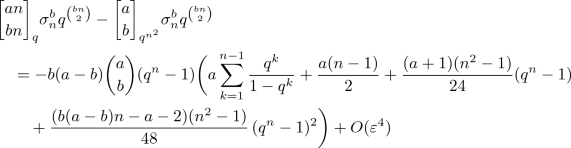as $$q=\zeta (1-\varepsilon )\rightarrow \zeta$$ radially. This means that the difference of both sides is divisible by $$(q-\zeta )^4$$ for any n-th primitive root of unity $$\zeta$$, hence by $$\Phi _n(q)^4$$. The latter property is equivalent to the congruence (1.6). $$\square$$

### Proof

We first use Lemma 2.1 with $$n=1$$:as $$q\rightarrow 1$$. Now, take $$n>1$$ arbitrarily and apply this relation with q replaced with $$q^n$$, where $$q=\zeta (1-\varepsilon )$$, $$0<\varepsilon <1$$ and $$\zeta$$ is a primitive n-th root of unity:as $$\varepsilon \rightarrow 0$$. At the same time, from Lemma 2.1, we have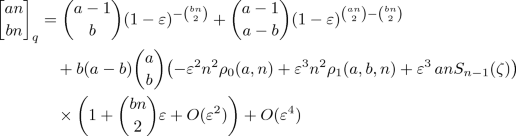as $$\varepsilon \rightarrow 0$$. Using

\begin{aligned} (1-\varepsilon )^N=1-N\varepsilon +\left( {\begin{array}{c}N\\ 2\end{array}}\right) \varepsilon ^2-\left( {\begin{array}{c}N\\ 3\end{array}}\right) \varepsilon ^3+O(\varepsilon ^4) \quad \text {as}~ \varepsilon \rightarrow 0 \end{aligned}

for $$N=-\left( {\begin{array}{c}bn\\ 2\end{array}}\right) +ab\left( {\begin{array}{c}n\\ 2\end{array}}\right)$$, $$\left( {\begin{array}{c}an\\ 2\end{array}}\right) -\left( {\begin{array}{c}bn\\ 2\end{array}}\right) -a(a-b)\left( {\begin{array}{c}n\\ 2\end{array}}\right)$$, $$-\left( {\begin{array}{c}bn\\ 2\end{array}}\right)$$ and $$\left( {\begin{array}{c}an\\ 2\end{array}}\right) -\left( {\begin{array}{c}bn\\ 2\end{array}}\right)$$ we deduce from the two expansions and Lemma 3.1 that, for $$q=\zeta (1-\varepsilon )$$,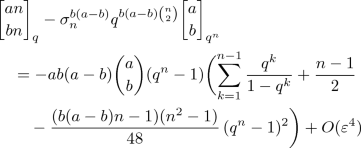as $$\varepsilon \rightarrow 0$$. This implies the congruence in (1.7). $$\square$$

## q-Rious Congruences

In this final part, we look at the binomial coefficients as particular instances of integral ratios of factorials, also known as Chebyshev–Landau factorial ratios. In the q-setting, these are defined by

\begin{aligned} D_n(q)=D_n(\varvec{a},\varvec{b};q)=\frac{[a_1n]!\cdots [a_rn]!}{[b_1n]!\cdots [b_sn]!}, \end{aligned}

where $$\varvec{a}=(a_1,\dots ,a_r)$$ and $$\varvec{b}=(b_1,\dots ,b_s)$$ are tuples of positive integers satisfying

\begin{aligned} a_1+\dots +a_r=b_1+\dots +b_s \end{aligned}
(5.1)

and

\begin{aligned} \lfloor a_1x\rfloor +\dots +\lfloor a_rx\rfloor \ge \lfloor b_1x\rfloor +\dots +\lfloor b_sx\rfloor \quad \text {for all}~ x>0 \end{aligned}
(5.2)

(see, for example, ), $$\lfloor \,\cdot \,\rfloor$$ denotes the integer part of a number. Then $$D_n(q)\in {\mathbb {Z}}[q]$$ are polynomials with values

\begin{aligned} D_n(1)=\frac{(a_1n)!\cdots (a_rn)!}{(b_1n)!\cdots (b_sn)!} \end{aligned}

at $$q=1$$, and the congruences (1.2) and (1.5) generalise as follows.

### Theorem 5.1

In the notation

\begin{aligned} c_i=c_i(\varvec{a},\varvec{b})=\left( {\begin{array}{c}a_1\\ i\end{array}}\right) +\dots +\left( {\begin{array}{c}a_r\\ i\end{array}}\right) -\left( {\begin{array}{c}b_1\\ i\end{array}}\right) -\cdots -\left( {\begin{array}{c}b_s\\ i\end{array}}\right) \quad \text {for}\; i=2,3, \end{aligned}

the congruences

\begin{aligned} D_n(q)&\equiv D_1(q^{n^2})-D_1(1)\frac{c_2\,(n^2-1)}{24}\,(q^n-1)^2\pmod {\Phi _n(q)^3} \end{aligned}
(5.3)

and

\begin{aligned} D_n(q)&\equiv \sigma _n^{c_2}q^{c_2\left( {\begin{array}{c}n\\ 2\end{array}}\right) }D_1(q^n)+D_1(1)\frac{(c_2+c_3)\,(n^2-1)}{12}\,(q^n-1)^2\pmod {\Phi _n(q)^3} \end{aligned}
(5.4)

are valid for any $$n\ge 1$$.

Observe that when $$n=p>3$$ and $$q\rightarrow 1$$, one recovers from any of these two congruences

\begin{aligned} D_p(1)\equiv D_1(1)\pmod {p^3}, \end{aligned}

of which (1.1) is a special case. Furthermore, it is tempting to expect that these two families of q-congruences may be generalised even further in the spirit of Theorems 1.1 and 1.2, and that the polynomials $$D_n(q)$$ satisfy the q-Gauss relations from . We do not pursue this line here.

### Proof of Theorem 5.1

Though the congruences (5.3) and (5.4) are between polynomials rather than rational functions, we prove the theorem without the assumption (5.2): in other words, the congruences remain true for the rational functions $$D_n(q)$$ provided that the balancing condition (5.1) (equivalently, $$c_1(\varvec{a},\varvec{b})=0$$ in the above notation for $$c_i$$) is satisfied. In turn, this more general statement follows from its validity for particular cases

\begin{aligned} D_n(q)=\frac{[an]!}{[bn]!\,[(a-b)n]!} \quad \text {and}\quad \tilde{D}_n(q)=\frac{[bn]!\,[(a-b)n]!}{[an]!} \end{aligned}

by induction (on $$r+s$$, say). Indeed, the inductive step exploits the property of both (5.3) and (5.4) to imply the congruence for the product

\begin{aligned} D_n(\varvec{a},\varvec{b};q)D_n(\tilde{\varvec{a}},\tilde{\varvec{b}};q) \end{aligned}

whenever it is already known for the individual factors; we leave this simple fact to the reader and only discuss its other appearance when dealing with $${\tilde{D}}_n(q)$$ below. Notice that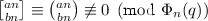, so that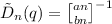is well-defined modulo of any power of $$\Phi _n(q)$$.

For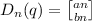, we have $$c_2=b(a-b)$$ and $$c_2+c_3=ab(a-b)/2$$; hence, (5.3) and (5.4) follow from (1.2) and (1.5), respectively.

Turning to $$q=\zeta (1-\varepsilon )$$, where $$0<\varepsilon <1$$ and $$\zeta$$ is a primitive n-th root of unity, write the congruences (1.2) and (1.5) as the asymptotic relation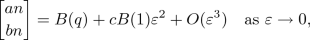in which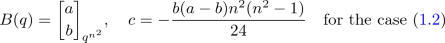andThenbecause we have $$B(q)=B(1)+O(\varepsilon )$$ as $$\varepsilon \rightarrow 0$$ for our choices of B(q). The resulting expansion implies the truth of (5.3) and (5.4) for $$\tilde{D}_n(q)=D_n((b,a-b),(a);q)$$ in view of

\begin{aligned} c_i((b,a-b),(a))=-c_i((a),(b,a-b)) \quad \text {for}~ i=2,3. \end{aligned}

As explained above, this also establishes the general case of (5.3) and (5.4).$$\square$$

For related Lucas-type congruences satisfied by the q-factorial ratios $$D_n(q)$$, see .

## References

1. Adamczewski, B., Bell, J.P., Delaygue, É., Jouhet, F.: Congruences modulo cyclotomic polynomials and algebraic independence for $$q$$-series. Sém. Lothar. Combin. 78B, #A54 (2017)

2. Andrews, G.E.: $$q$$-Analogs of the binomial coefficient congruences of Babbage, Wolstenholme and Glaisher. Discrete Math. 204(1-3), 15–25 (1999)

3. Andrews, G.E., Askey, R., Roy, R.: Special Functions. Cambridge University Press, Cambridge (1999)

4. Gorodetsky, O.: $$q$$-Congruences, with applications to supercongruences and the cyclic sieving phenomenon. Intern. J. Number Theory 15(9), 1919–1968 (2019)

5. Guo, V.J.W., Zudilin, W.: A $$q$$-microscope for supercongruences. Adv. Math. 346, 329–358 (2019)

6. Meštrović, R.: Wolstenholme’s theorem: its generalizations and extensions in the last hundred and fifty years (1862–2012). arXiv:1111.3057 (2011)

7. Pan, H.: Factors of some lacunary $$q$$-binomial sums. Monatsh. Math. 172(4), 387–398 (2013)

8. Straub, A.: A $$q$$-analog of Ljunggren’s binomial congruence. In: DMTCS Proceedings: 23rd International Conference on Formal Power Series and Algebraic Combinatorics (FPSAC 2011), Discrete Math. Theor. Comput. Sci. Proc., AO, pp. 897–902. Assoc. Discrete Math. Theor. Comput. Sci., Nancy (2011)

9. Straub, A.: Supercongruences for polynomial analogs of the Apéry numbers. Proc. Amer. Math. Soc. 147(3), 1023–1036 (2019)

10. Warnaar, S.O., Zudilin, W.: A $$q$$-rious positivity. Aequationes Math. 81(1-2), 177–183 (2011)

## Acknowledgements

I would like to thank Armin Straub for encouraging me to complete this project and for the supply of available knowledge on the topic. I am grateful to one of the referees whose feedback was terrific and helped me improving the exposition. Further, I thank Victor Guo for valuable comments on parts of this work.

Authors